### 7.4 Examples

#### 7.4.1 Aeroacoustic Wave Propagation

In this section we explain how to set up a simple simulation of aeroacoustics in Nektar++. We will study the propagation of an acoustic wave in the simple case where the base flow is ui = 0,p = p = 106,ρ = ρ0 = 1.204. The geometry consists of 64 quadrilateral elements, as shown in Fig. 7.1.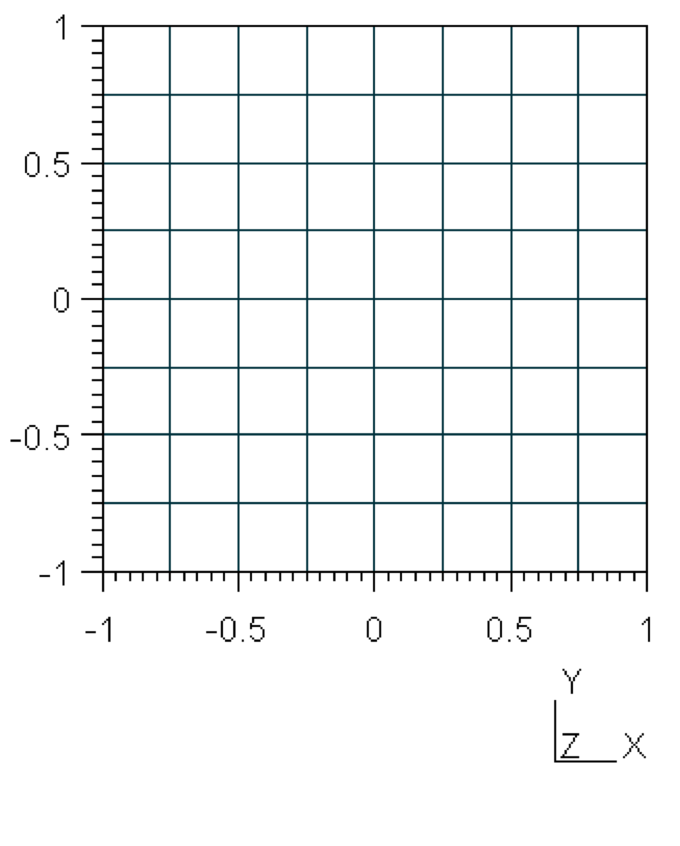Figure 7.1: Geometry used for the example case of modelling propagation of acoustic waves where ui = 0,p = p = 106,ρ = ρ0 = 1.204

##### 7.4.1.1 Input file

We require a discontinuous Galerkin projection and use an explicit fourth-order Runge-Kutta time integration scheme. We therefore set the following solver information:

1<I PROPERTY="EQType" VALUE="APE"/>
2<I PROPERTY="Projection" VALUE="DisContinuous"/>
3<I PROPERTY="TimeIntegrationMethod"  VALUE="ClassicalRungeKutta4"/>
4<I PROPERTY="UpwindType"  VALUE="APEUpwind"/>

To maintain numerical stability we must use a small time-step. The total simulation time is 150 time units. Finally, we set the density, heat ratio and ambient pressure.

1<P> TimeStep       = 0.00001             </P>
2<P> NumSteps       = 150                 </P>
3<P> FinTime        = TimeStep*NumSteps   </P>
4<P> Rho0           = 1.204               </P> <!-- Incompressible density -->
5<P> Gamma          = 1.4                 </P> <!-- Ratio of specific heats -->
6<P> Pinfinity      = 100000              </P> <!-- Ambient pressure -->

Let us note that to solve efficiently this problem a discontinuous Garlerkin approach was used. The system is excited via the initial conditions putting a Gaussian pulse for pulse fluctuations. Finally, it is necessary to specify the base flow and the eventual source terms using the following functions:

1<FUNCTION NAME="Baseflow">
2    <E VAR="u0"   VALUE="0" />
3    <E VAR="v0"   VALUE="0" />
4    <E VAR="p0"   VALUE="Pinfinity" />
5    <E VAR="rho0" VALUE="Rho0" />
6</FUNCTION>
7
8<FUNCTION NAME="Source">
9    <E VAR="S"  VALUE="0" />
10</FUNCTION>
11
12<!-- Gaussian pulse located at the origin -->
13<FUNCTION NAME="InitialConditions">
14    <E VAR="p" VALUE="100*exp(-32*(((x)*(x))+((y)*(y))))" />
15    <E VAR="u" VALUE="0" />
16    <E VAR="v" VALUE="0" />
17</FUNCTION>

##### 7.4.1.2 Running the code
APESolver Test_pulse.xml

##### 7.4.1.3 Results

Fig. 7.2 shows the pressure profile at different time steps, showing the acoustic propagation.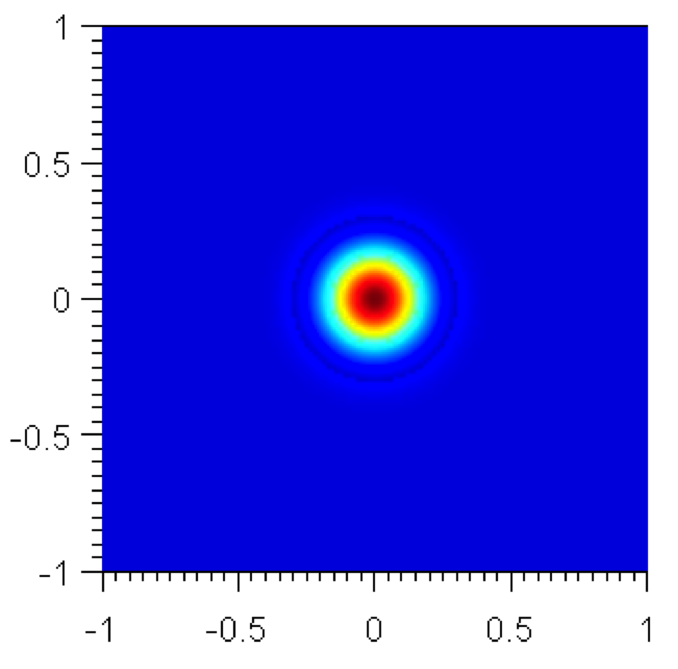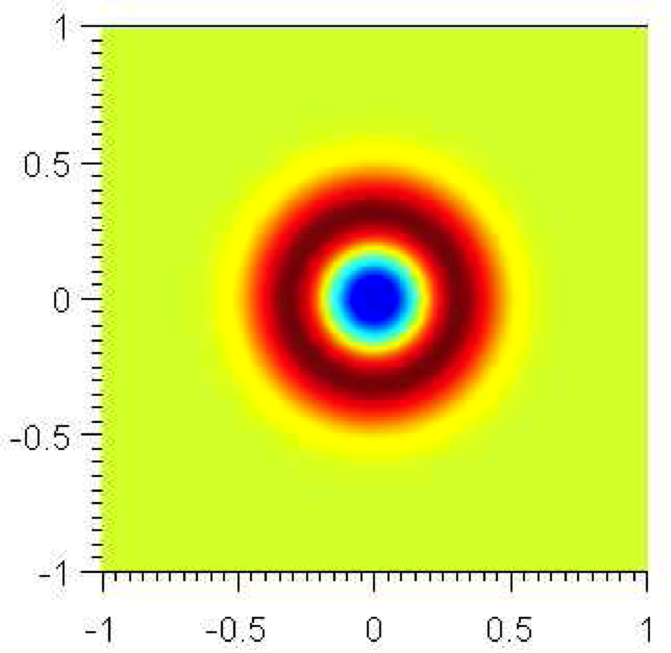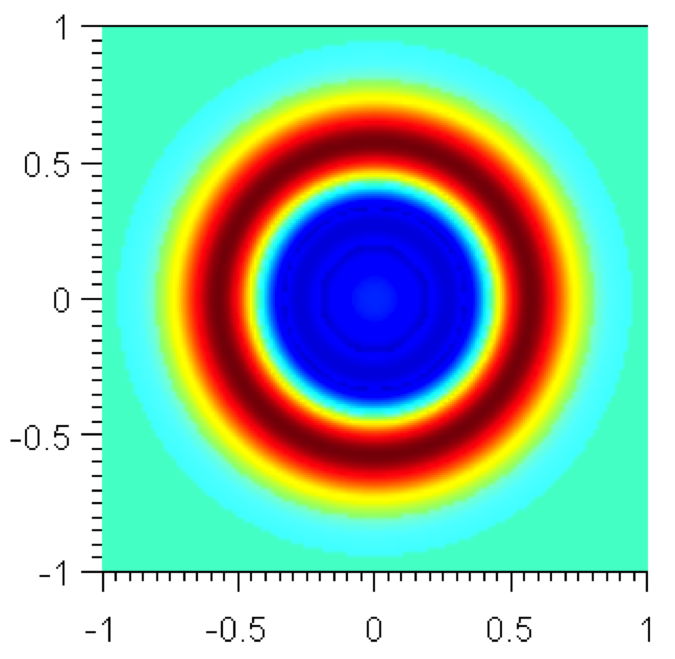Figure 7.2:

It is possible to show the profile of the pressure perturbations with respect to the spatial coordinate. The pressure fluctuations, that are concentrated in a specific locations at the beginning (as specified by the initial conditions), propagate with time and for sufficiently large time the decay is exponential as predicted by literature .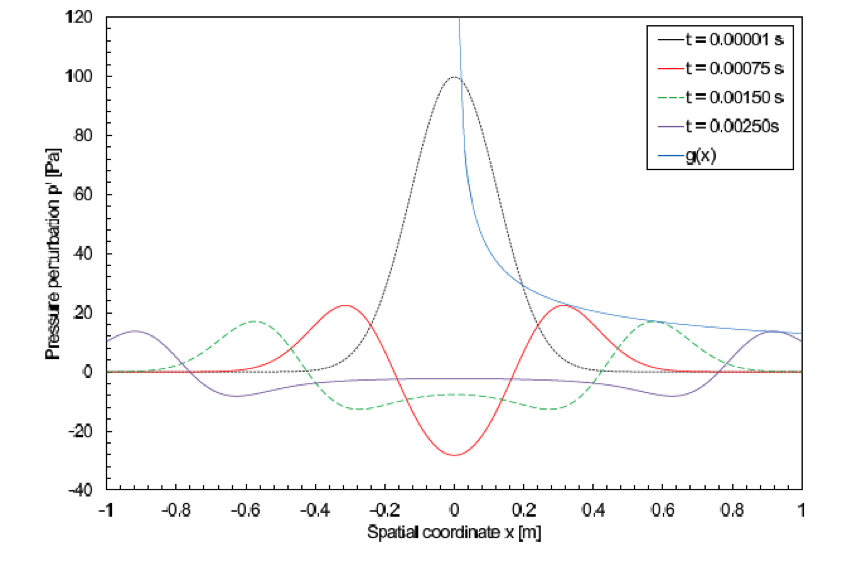Figure 7.3: